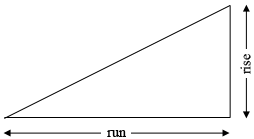SEARCH HOMEMath Central Quandaries & QueriesQuestion from Ashiq: What is 1% slope if length 101 feet?Hi,The slope of a line is given by

$\mbox{slope } = \frac{\mbox{rise}}{\mbox{run}}.$

You have a slope of 0.01 and a run of 101 feet. Solve for the rise.

PennyMath Central is supported by the University of Regina and the Imperial Oil Foundation.